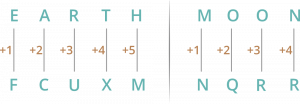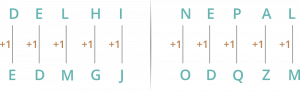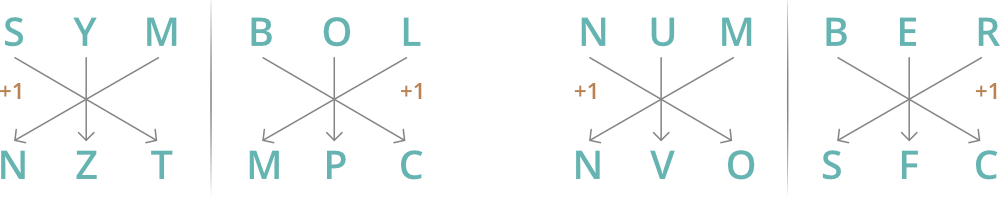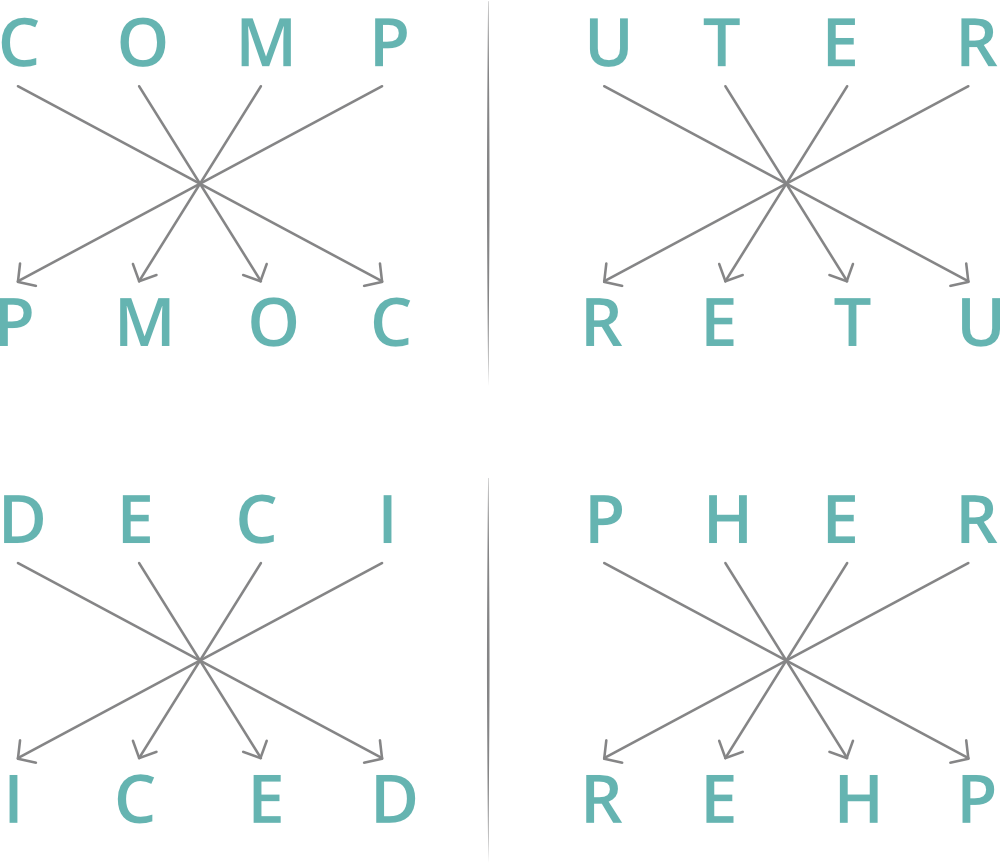# Coding-Decoding

CODING-DECODING is an important part of Logical reasoning section in all aptitude related examinations.
Coding is a process used to encrypt a word, a number in a particular code or pattern based on some set of rules.
Decoding is a process to decrypt the pattern into its original form from the given codes.

Letter Coding In this type of questions, alphabets of a word are replaced by some other alphabets according to specific rule to form code.

Number Coding In this type of questions, a word is replaced by certain numbers according to some specific rule.

## Sample Problems –

Question 1: If EARTH is written as FCUXM in a certain code. How is MOON written in that code?
Solution :Question 2: If DELHI is written as EDMGJ in a certain code. How is NEPAL written in that code?
Solution :Question 3: If SYMBOL is written as NZTMPC is a certain code. How is NUMBER written in that code?
Solution : Acc. to questionQuestion 4: In a certain code, COMPUTER is written as PMOCRETU, how is DECIPHER written in that code?
Solution : Acc. to questionQuestion 5: In a certain code, NEWYORK is written as 111, how is NEWJERSEY written in that code?
Solution : In NEWYORK
N = 14, E = 5, W = 23, Y = 25, O = 15, R = 18, K = 11
Total = 14 + 5 + 23 + 25 + 15 + 18 + 11 = 111
In NEWJERSEY
Total = 14 + 5 + 23 + 10 + 5 + 18 + 19 + 5 + 25
= 124

Question 6: In a certain code, HARYANA is written as 8197151, how is DELHI written in that code?
Solution : We used the number of alphabets here.
H = 8
A = 1
R = 18 = 1+8 = 9
Y = 25 = 2+5 = 7
For DELHI
D = 4
E = 5
L = 12 = 1+2 = 3
H = 8
I = 9
Hence, DELHI is written as 45389.

Question 7: In a certain code BOMB is written as 5745 and BAY is written as 529, how is BOMBAY written in that code?
Solution : Acc. to question
Use the numbers to relate the words

```      B O M B      B A Y      ->          B O M B A Y
5 7 4 5      5 2 9                  5 7 4 5 2 9

```

Practice Problems:

Q1: If in a certain language, MADRAS is coded as NBESBT, how is BOMBAY coded in that code?

(a) CPNCBX (6) CPNCBZ (c) CPOCBZ (d) CQOCBZ (e) None of these

Q2: In a certain code, TRIPPLE is written as SQHOOKD. How is DISPOSE written in that code?

(a) CHRONRD (6) DSOESPI (c) ESJTPTF (d) ESOPSID (e) None of these

Q3: If in a code language. COULD is written as BNTKC and MARGIN is written as LZQFHM, how will MOULDING be written in that code?

(a) CHMFINTK (6) LNKTCHMF (c) LNTKCHMF (d) NITKHCMF (e) None of these

Q4: In a certain code, MONKEY is written as XDJMNL. How is TIGER written in that code?

(a) QDFHS (6) SDFHS (c) SHFDQ (d) UJHFS (e) None of these

Q5: If FRAGRANCE is written as SBHSBODFG, how can IMPOSING be written?

(a) NQPTJHOJ (6) NQPTJOHI (c) NQTPJOHJ (d) NQPTJOHJ (c) None of these

Q1: (b), Q2: (a), Q3: (c), Q4: (a), Q5: (d)

My Personal Notes arrow_drop_upCheck out this Author's contributed articles.

If you like GeeksforGeeks and would like to contribute, you can also write an article using contribute.geeksforgeeks.org or mail your article to contribute@geeksforgeeks.org. See your article appearing on the GeeksforGeeks main page and help other Geeks.

Please Improve this article if you find anything incorrect by clicking on the "Improve Article" button below.

Improved By : mansi_modi

Article Tags :

11

Please write to us at contribute@geeksforgeeks.org to report any issue with the above content.